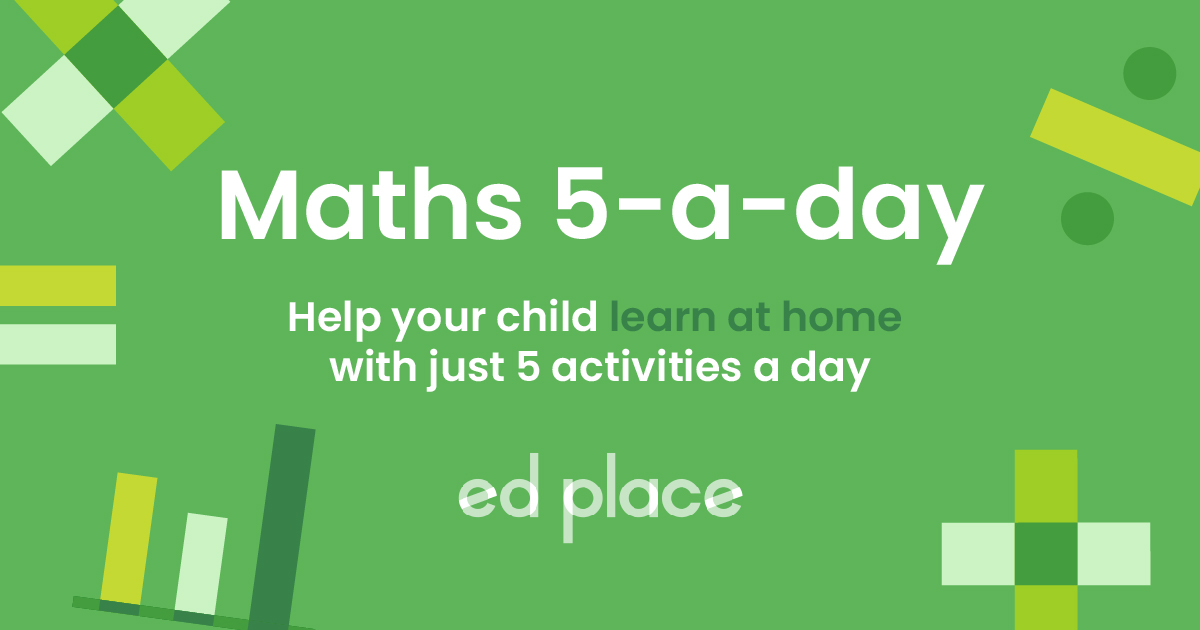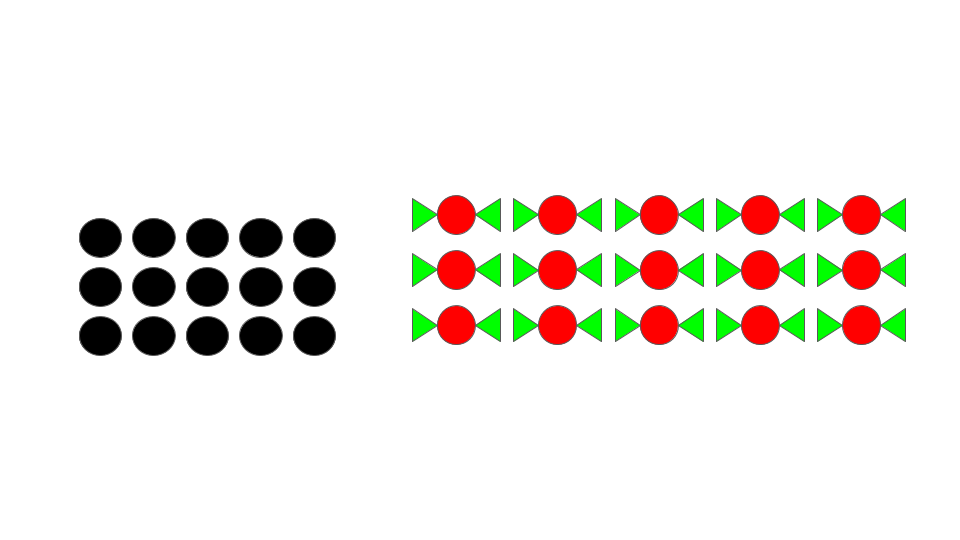# EdPlace's Year 2 Home Learning Maths Lesson: Multiplication

Looking for short lessons to keep your child engaged and learning? Our experienced team of teachers have created English, maths and science lessons for the home, so your child can learn no matter where they are.  And, as all activities are self-marked, you really can encourage your child to be an independent learner. Get them started on the lesson below and then jump into our teacher-created activities to practice what they've learnt. We've recommended five to ensure they feel secure in their knowledge - 5-a-day helps keeps the learning loss at bay (or so we think!).

Are they keen to start practising straight away? Head to the bottom of the page to find the activities.

Now...onto the lesson!

Key Stage 1 Statutory Requirements for Maths
Year 2 Students should be able to solve problems involving multiplication and division, using arrays

# Multiplication often sounds a lot more complicated than it actually is, doesn't it?

Year 2 has a big focus on children understanding what multiplication means alongside learning to recall the times tables. It is important that the children have a clear understanding that multiplication means ‘groups of’, for example, 5 &times; 4 is 5 groups of 4. Using arrays is a great way of consolidating this understanding. Children can also use arrays to help them answer word problems that involve multiplication.

EdPlace are here to help you understand how to use this method using a step - by - step approach so you will know what the teachers are talking about

1) Understand exactly what is meant by solving multiplication problems using arrays.

2) Apply it to both multiplication word problems and solving any multiplication questions.

3) Explain to you how they completed a multiplication question!

## Step 1 - Understand key terminology

Just like in school, it is so important that the children understand the vocabulary used in each lesson. So here we will go through the meaning of the vocabulary for this lesson.

Multiplication - This is repeated addition. You could think of it as ‘lots of’. So 3 multiplied by 5 means 3 lots of 5 which is the same as 5 + 5 + 5.

Array - A pictorial representation of multiplication. For example, 6 × 2 will show 6 rows of 2. This could be using pictures or simply dots.## Step 2 - Example 1

We need to be able to carefully read a word problem to work out that if it needs multiplication to work out the answer.  First, read the word problem slowly and then read it again to ensure you have understood what it is asking you and what the multiplication sentence will be. Here is the word problem we will be using for our example:

Sophie got some sweets after school for her and her friends. Each bag had 5 sweets in and she got 3 bags. How many sweets were there altogether?

This is a multiplication question. 3 bags each have 5 sweets and you are asked how many sweets there are altogether.  So it is asking for 3 lots of 5 so you will write this as 3 &times; 5 = (think of the &times; sign as meaning ‘lots of’) You can then use the array to help you work out the answer. If it is not clear to your child that it is a multiplication question then they can also use an array here to work out what they need to do, which is explained in the next step.

## Step 3 - Get to grips with the long multiplication method

If your child was unable to derive the number sentence 3 × 5 = from the question they can use an array to help them.

We know each bag has 5 sweets and that there are 3 bags. So we need to make a row of 5 sweets as we know that is how many there are in each bag. Then we need to repeat this row so that there are 3 rows as we know there are 3 lots of these 5 sweets. You could draw sweets in your array or just use dots. Below is an example of each.If your child worked out that the multiplication sentence was 3 × 5 = but needs support working out the answer then they can now draw the array to help them. Thinking of 3 × 5 = as 3 lots of 5 which is 3 rows of 5. Once they have drawn the array they can count how many sweets/dots there are altogether and find their answer. So the answer here is 15.

## Step 4 - Putting it into practise...

You could now apply the above to work out the questions below, starting with multiplication questions and moving onto word problems:

1. 7 × 2 =

2. 4 × 5 =

3. There are 9 packets of crayons. Each packet has 5 crayons in. How many crayons are there altogether?

4. A boy keeps all his footballs in big bags. He has 3 footballs in each bag and he has 6 bags. How any footballs altogether?

5. Sophie has some flowers in her bedroom. Each flower has 6 petals. There are 4 flowers. How many petals altogether?

## Step 5 - Give it a go...

Now that you’ve covered this lesson together, why not put this to the test and assign your child the following multiplication activities in this order? All activities are created by teachers and automatically marked. Plus, with an EdPlace subscription, we can automatically progress your child at a level that's right for them. Sending you progress reports along the way so you can track and measure progress, together - brilliant!

1. 14

2. 20

3. 45 crayons

4. 6 × 3 = 18 footballs

5. 4 × 6 = 24 petals

Keep going! Looking for more activities, different subjects or year groups?

Click the button below to view the EdPlace English, maths, science and 11+ activity library